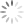月泉的博客

# 手把手带你分析Class字节码文件

``````package org.yuequan.klass;

public class Foo{
private int m;

public int inc(){
return m + 1;
}
}
``````

Java虚拟机规范对Class文件结构做了严格的规定，其中的字节严格的按顺序紧密的排在一起，中间没有任何的分隔符和其它数据``````// index 1
tag = 10
class_index = 4
name_and_type_index = 15
``````

``````// index 2
tag = 9
class_index = 3
name_and_type_index = 16
``````

``````// index 3
tag = 7
name_index = 17
``````

`````` // index 4
tag = 7
name_index = 18
``````

``````// index  5
tag = 1
length = 1
bytes = ['m'] // bytes = [6D]  6D = 109 => UTF8 => m
``````

``````// index 6
tag = 1
length = 1
bytes = ['I'] // bytes = ['49'] 49 => 73 => UTF8 => I
``````

``````// index 6
tag = 1
length = 6
//  23333 很长于是注释写这里
// [0x3C, 0x69, 0x6E, 0x69, 0x74, 0x3E]
// to UTF8
// ['<', 'i', 'n', 'i', 't', '>']
// bytes.toString() => "<init>"
bytes = ['<', 'i', 'n', 'i', 't', '>']
``````

``````// index 8
tag = 1
length = 3
bytes = ['(', ')', 'V'] //不再重复转换步骤拉
``````

``````// index 9
tag = 1
length = 4
bytes = ['C', 'o', 'd', 'e']
``````

``````// index 10
tag = 1
length = 15
bytes = ['L', 'i', 'n', 'e', 'N', 'u', 'm', 'b', 'e', 'r', 'T', 'a', 'b', 'l', 'e']
``````

``````// index 11
tag = 1
length = 3
bytes = ['i', 'n', 'c']
``````

``````// index 12
tag = 1
length = 3
bytes = ['(', ')', 'I']
``````

``````// index 13
tag = 1
length = 10
bytes = ['S', 'o', 'u', 'r', 'c', 'e', 'F', 'i', 'l', 'e'] //SourceFile
``````

``````// index 14
tag = 1
length = 8
bytes = ['F', 'o', 'o', '.', 'j', 'a', 'v', 'a'] // Foo.java
``````

``````// index 15
tag = 12
name_index =  7
descriptor_index = 8
``````

``````// index 16
tag = 12
name_index = 5
descriptor_index = 6
``````

``````// index 17
tag = 1
length = 21
bytes = ['o', 'r', 'g', '/', 'y', 'u', 'e', 'q', 'u', 'a', 'n', '/', 'k', 'l', 'a', 's', 's', '/', 'F', 'o', 'o']
``````

``````// index 18
tag = 1
length = 16
bytes = ['j', 'a', 'v', 'a', '/', 'l', 'a', 'n', 'g', '/', 'O', 'b', 'j', 'e', 'c', 't']
``````大致分析出来的内容用思维导图整理了一下，最终整合一波

``````// index 1
// class_index = 4  name_and_type = 15
Methodref  java/lang/Object.<init>

// index 2
// class_index = 3  name_and_type = 16
Fieldref org/yuequan/klass/Foo.m

// index 3
// name_index = 17
Class org/yuequan/klass/Foo

// index 4
// name_index = 18
Class java/lang/Object

// index 5
Utf8 m

// index 6
Utf8 I

// index 7
Utf8 <init>

// index 8
Utf8 ()V

// index 9
Utf8 Code

// index 10
Utf8 LineNumberTable

// index 11
Utf8 inc

// index 12
Utf8 ()I

// index 13
Utf8 SourceFile

// index 14
Utf8 Foo.java

// index 15
// name_index = 7 descriptor_index = 8
NameAndType  <init>&()V

// index 16
// name_index = 5 descriptor_index = 6
NameAndType m&I

// index 17
Utf8 org/yuequan/klass/Foo

// index 18
Utf8 java/lang/Object
``````

``````access_flag = ACC_PUBLIC, ACC_SUPER
``````接下来是接口此类没有实现接口数量为0